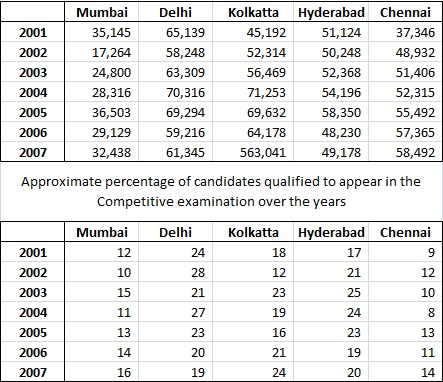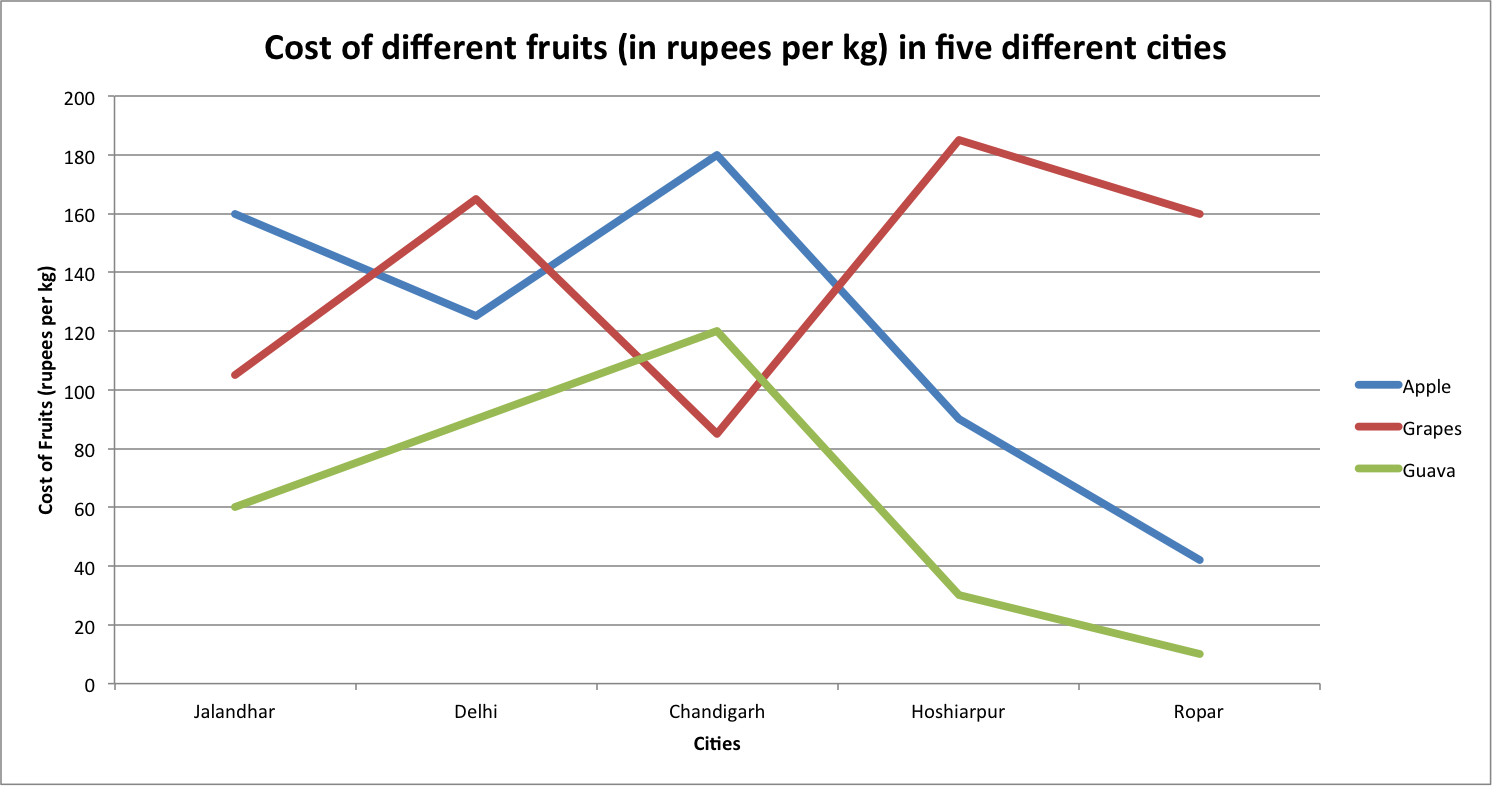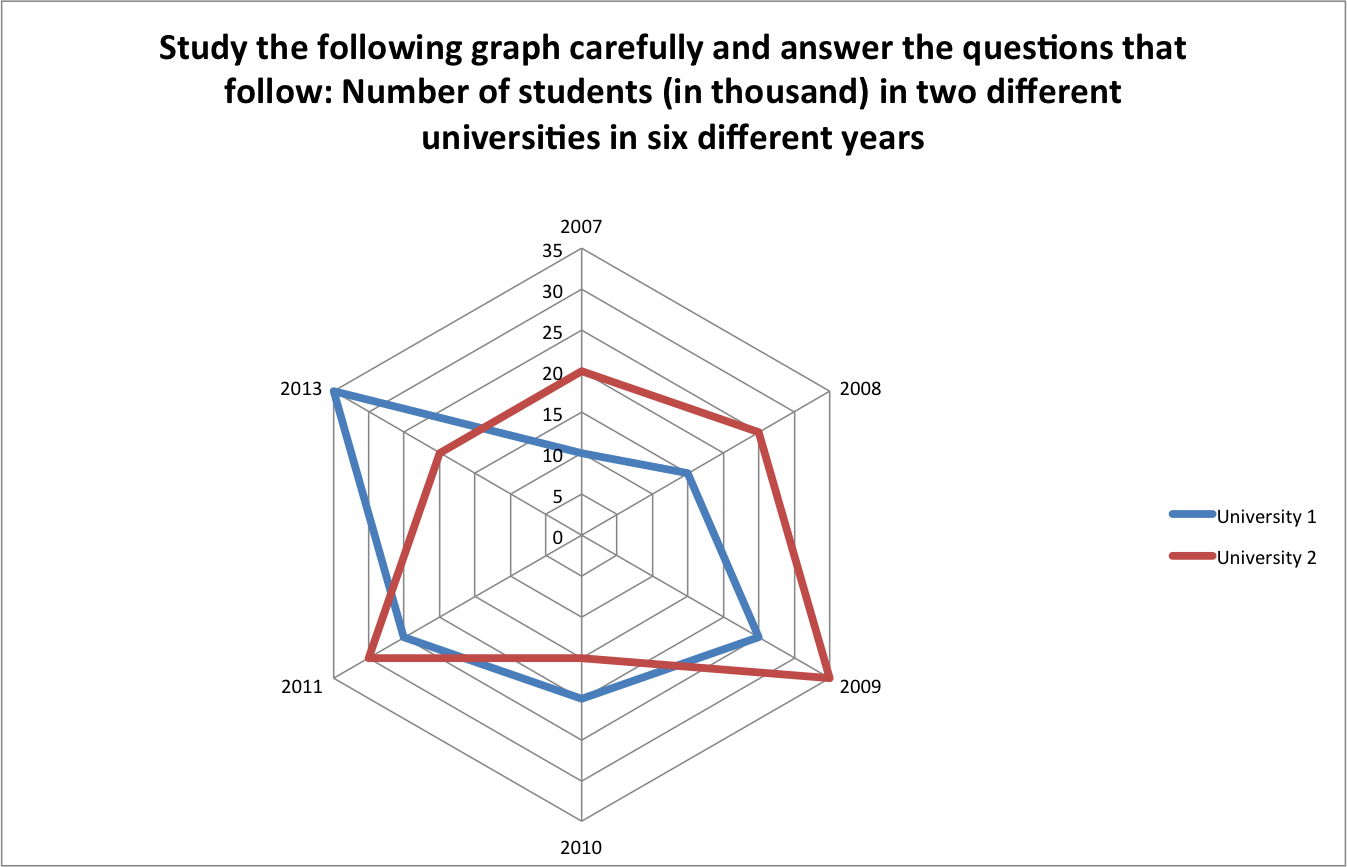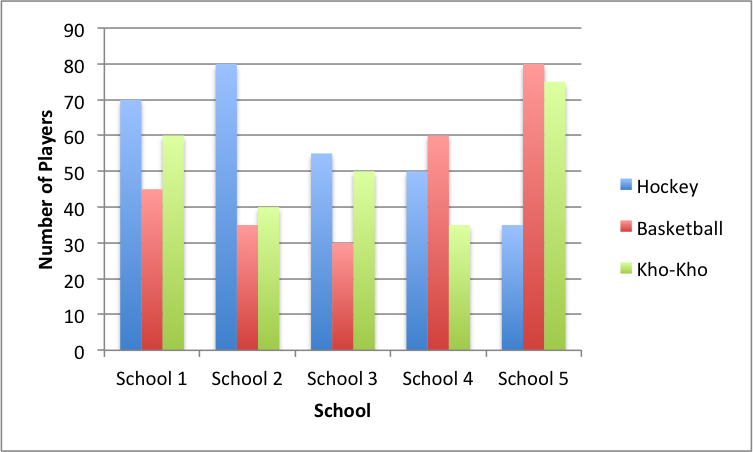0
727

Data Interpretation Questions For IBPS Clerk Set 3 PDF

Download important  Data Interpretation Questions PDF based on previously asked questions in IBPS Clerk and other Banking Exams. Practice Data Interpretation Questions and Answers for IBPS Clerk Exam.

Take Free IBPS Clerk Mock Test

Go to Free Banking Study Material (15,000 Solved Questions)

Instructions

Direction: Study the following tables carefully and answer the questions given below:Number of Candidates appeared in a Competitive Examination from five centres over the years
Question 1: In which of the following years was the difference in number of candidates appeared from Mumbai over the previous year the minimum ?

a) 2004

b) 2006

c) 2007

d) 2002

e) None of these

Question 2: In which of the following years was the number of candidates qualified from Chennai, the maximum among the given years ?

a) 2007

b) 2006

c) 2005

d) 2003

e) None of these

Question 3: Approximately what was the total number of candidates qualified from Delhi in 2002 and 2006 together ?

a) 27250

b) 25230

c) 30150

d) 28150

e) 26250

Question 4: Approximately how many candidates appearing from Kolkata in 2004 qualified in the competitive examination ?

a) 13230

b) 13540

c) 15130

d) 15400

e) 19240

Question 5: Approximately what was the difference between the number of candidates qualified from Hyderabad in 2001 and 2002 ?

a) 1680

b) 2440

c) 1450

d) 2060

e) 1860

Instructions

Directions: Study the following graph carefully and answer the questions that follow:Question 6: In which city is the difference between the cost of 1 kg of apple and the cost of one kg of guava the second lowest?

a) Jalandhar

b) Delhi

c) Chandigarh

d) Hoshiarpur

e) Ropar

Question 7: The cost of 1 kg of guava in Jalandhar is approximately What per cent of the cost of 2 kg of grapes in Chandigarh?

a) 66

b) 24

c) 28

d) 34

e) 58

Question 8: What total amount will Ram pay to the Shopkeeper for purchasing 3 kg of apples and 2 Kg. of guavas in Delhi?

a) Rs 530

b) Rs 450

c) Rs 570

d) Rs 620

e) Rs 490

Question 9: Ravinder had to purchase 45 kg of grapes from Hoshiarpur. The Shopkeeper gave him a discount of 4% per kg. What amount did he pay to the Shopkeeper after the discount?

a) Rs 7992

b) Rs 8104

c) Rs 8340

d) Rs 8550

e) Rs 8410

Question 10: What is the ratio of the cost of 1kg of apples from Ropar to the cost of 1kg. of grapes from Chandigarh?

a) $3 : 2$

b) $2 : 3$

c) $2^2 : 3^2$

d) $4^2 : 9^2$

e) $9^2 : 4^2$

Instructions

Directions:Question 11: What was the difference between the number of students in University-1 in the year 2010 and the number of students in University-2 in the year 2013?

a) Zero

b) 5,000

c) 15,000

d) 10,000

e) 1,000

Question 12: What is the sum of the number of students in University-1 in the year 2007 and the number of students in University-2 in the year 2011 together?

a) 50,000

b) 55,000

c) 45,000

d) 57,000

e) 40,000

Question 13: If 25% of the students in univeristy-2 in the year 2010 were females, what was the number of male students in University-2 in the same year?

a) 11,250

b) 12,350

c) 12,500

d) 11,500

e) 11,750

Question 14: What was the percent increase in the number of students in University-1 in the year 2011 as compared to the previous year?

a) 135

b) 15

c) 115

d) 25

e) 35

Question 15: In which year was the difference between the number of students in University- 1 and the number of students in University-2 the highest?

a) 2008

b) 2009

c) 2010

d) 2011

e) 2013

Instructions

Directions: Study the graph carefully to answer the questions that follow. Number of players in three different games in five different schoolsQuestion 16: What is the total number of players participating in Hockey from all the five schools together?

a) 324

b) 288

c) 342

d) 284

e) 248

Question 17: What is the ratio of the number of players participating in Basketball from School-1 to the number of players participating in Kho-Kho from School-3?

a) 5 : 7

b) 7 : 9

c) 7 : 8

d) 9 : 7

e) 5 : 8

Question 18: In which school is the number of players participating in Hockey and Basketball together the second lowest?

a) School-1

b) School-2

c) School-3

d) School-4

e) School-5

Question 19: The number of players participating in Kho-Kho from School-4 is what percent of the number of players participating in Hockey from School-2?

a) 42

b) 48

c) 36

d) 40

e) 60

Question 20: 25% of the number of players participating in Hockey from School-5 are females. What is the number of the Hockey players who are males in School-5?

a) 15

b) 18

c) 30

d) 21

e) 27

The drastic fall of the number of candidates from 2001 (35,145) to 2002 (17,264) is 17,881.
In every other year (except a smaller drop from 2005-2006), the number of candidates has increased from one year to the next.

Hence, the correct answer is 2002

In 2007, the highest number of candidates appeared for the exam from Chennai and the pass percentage is also the highest compared to the other years. So, the number of candidates from Chennai who passed in the exam is the highest in 2007.

The number equals 14%*58492 = 8189

Number of candidates who qualified = 28% of 58248 + 20% of 59216 = 28150 (approx.)

Number of candidates who qualified from Kolkata in 2004 = 19% of 71253 = 13538 = 13540 (approx.)

Number of candidates who qualified from Hyderabad in 2001 = 17% of 51124 = 8691.08

Number of candidates who qualified from Hyderabad in 2002 = 21% of 50248 = 10552.08

Difference = 1860 (approx.)

Difference in cost of 1 kg pf apple and 1 kg of guava in Jalandhar = 160 – 60 = 100

Difference in cost of 1 kg pf apple and 1 kg of guava in Delhi = 125 – 90 = 35

Difference in cost of 1 kg pf apple and 1 kg of guava in Chandigarh = 180 – 120 = 60

Difference in cost of 1 kg pf apple and 1 kg of guava in Hoshiarpur = 90 – 30 = 60

Difference in cost of 1 kg pf apple and 1 kg of guava in Ropar = 40 – 10 = 30

=> Second lowest difference in Delhi

Cost of 1kg of Guava in Jalandhar = 60

Cost of 2kg of grapes in Chandigarh = 180

Required percentage = $\frac{60}{180}*100$ = 33.33 => 34 (Approx)

In Delhi, 3kg of apples = 130*3 = 390

2 kg of guava = 2*90 = 180

Total cost = 570

Cost of grapes per 45kg in Hoshiarpur = 45 * 185 = 8325

4% discount => 0.96 * 8325 = Rs 7992

Ratio of 1 kg of apples in Ropar to 1 kg of grapes in chandigarh = 40 : 90 = 4:9

Hence, option C is the answer.

Number of students in university 1 in 2010 = 20000

Number of students in university 2 in 2012 = 20000

Difference = 0

Number of students in university 1 in the year 2007 = 10000

Number of students in university 2 in the year 2011 = 30000

Sum  = 40000

Number of students in university 2 in 2010 = 15000

25% female => 75% male => 75*15000/100 = 11250

Number of students in university 1 in 2011 = 25000

Number of students in university 1 in 2010 = 20000

% increase = $\frac{5000}{20000}*100$ = 25%

According to the figure, the biggest gap between number of students in each of the universities is seen in 2013. Hence the difference is maximum in 2013.

Total Number of Hockey players = 70 + 80 + 55 + 50 + 35 = 290

Here, the closest option to 290 is 288. Hence the answer is 288.

Number of students participating in basketball from school 1 = 45

Number of students participating in kho-kho from school 3 = 50

Ratio = 9 : 10

The nearest ratio to 9:10 among the given options is 7:8. Hence the answer is 7:8

Students in school 1 who play either Hockey or Basketball = 70+45 = 115

Students in school 2 who play either Hockey or Basketball = 80+35 = 115

Students in school 3 who play either Hockey or Basketball = 55+30 = 85

Students in school 4 who play either Hockey or Basketball = 50+60 = 110

Students in school 5 who play either Hockey or Basketball = 35+80 = 115

Clearly school 4 has the second lowest number of students who play either hockey or basketball.

Number of school 4 students who play kho-kho = 35

Number of school 2 students who play hockey = 80

Required percentage = $\frac{35}{80}*100$ = 43.75

Nearest option is 42. Hence 42 is the answer.